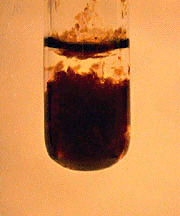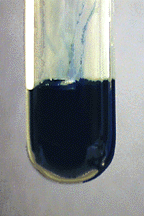# Characteristic Reactions of Iron (Fe³⁺)

$$\newcommand{\vecs}{\overset { \rightharpoonup} {\mathbf{#1}} }$$ $$\newcommand{\vecd}{\overset{-\!-\!\rightharpoonup}{\vphantom{a}\smash {#1}}}$$$$\newcommand{\id}{\mathrm{id}}$$ $$\newcommand{\Span}{\mathrm{span}}$$ $$\newcommand{\kernel}{\mathrm{null}\,}$$ $$\newcommand{\range}{\mathrm{range}\,}$$ $$\newcommand{\RealPart}{\mathrm{Re}}$$ $$\newcommand{\ImaginaryPart}{\mathrm{Im}}$$ $$\newcommand{\Argument}{\mathrm{Arg}}$$ $$\newcommand{\norm}{\| #1 \|}$$ $$\newcommand{\inner}{\langle #1, #2 \rangle}$$ $$\newcommand{\Span}{\mathrm{span}}$$ $$\newcommand{\id}{\mathrm{id}}$$ $$\newcommand{\Span}{\mathrm{span}}$$ $$\newcommand{\kernel}{\mathrm{null}\,}$$ $$\newcommand{\range}{\mathrm{range}\,}$$ $$\newcommand{\RealPart}{\mathrm{Re}}$$ $$\newcommand{\ImaginaryPart}{\mathrm{Im}}$$ $$\newcommand{\Argument}{\mathrm{Arg}}$$ $$\newcommand{\norm}{\| #1 \|}$$ $$\newcommand{\inner}{\langle #1, #2 \rangle}$$ $$\newcommand{\Span}{\mathrm{span}}$$$$\newcommand{\AA}{\unicode[.8,0]{x212B}}$$

• Most common oxidation states: +2, +3
• M.P. 1535º
• B.P. 2750º
• Density 7.87 g/cm3
• Characteristics: Iron is a gray, moderately active metal.

## Characteristic reactions of Fe²⁺ and Fe³⁺

The $$\ce{[Fe(H2O)6]^{3+}}$$ ion is colorless (or pale pink), but many solutions containing this ion are yellow or amber-colored because of hydrolysis. Iron in both oxidation states forms many complex ions.

### Aqueous Ammonia

Aqueous ammonia reacts with Fe(II) ions to produce white gelatinous $$\ce{Fe(OH)2}$$, which oxidizes to form red-brown $$\ce{Fe(OH)3}$$:

$\ce{Fe^{2+}(aq) + 2NH3(aq) + 3H2O(l) <=> Fe(OH)2(s) + 2NH4^{+}(aq)} \nonumber$Aqueous ammonia reacts with $$\ce{Fe(III)}$$ ions to produce red-brown $$\ce{Fe(OH)3}$$:

$\ce{Fe^{3+}(aq) + 3NH3(aq) + 3H2O(l) <=> Fe(OH)3(s) + 3NH4^{+}(aq)} \nonumber$Both precipitates are insoluble in excess aqueous ammonia. Iron(II) hydroxide quickly oxidizes to $$\ce{Fe(OH)3}$$ in the presence of air or other oxidizing agents.

### Sodium Hydroxide

Sodium hydroxide also produces $$\ce{Fe(OH)2}$$ and $$\ce{Fe(OH)3}$$ from the corresponding oxidation states of iron in aqueous solution.

$\ce{Fe^{2+}(aq) + 2OH^{-}(aq) <=> Fe(OH)2(s)} \nonumber$$\ce{Fe^{3+}(aq) + 3OH^{-}(aq) <=> Fe(OH)3(s)} \nonumber$Neither hydroxide precipitate dissolves in excess sodium hydroxide.

### Potassium Ferrocyanide

Potassium ferrocyanide will react with $$\ce{Fe^{3+}}$$ solution to produce a dark blue precipitate called Prussian blue:

$\ce{K^{+}(aq) + Fe^{3+}(aq) + [Fe(CN)6]^{4-}(aq) <=> KFe[Fe(CN)6](s)} \label{Prussian}$With $$\ce{Fe^{2+}}$$ solution, a white precipitate will be formed that will be converted to blue due to the oxidation by oxygen in air:

$\ce{2Fe^{2+}(aq) + [Fe(CN)6]^{4-}(aq) <=> Fe2[Fe(CN)6](s) } \nonumber$Many metal ions form ferrocyanide precipitates, so potassium ferrocyanide is not a good reagent for separating metal ions. It is used more commonly as a confirmatory test.

### Potassium Ferricyanide

Potassium ferricyanide will give a brown coloration but no precipitate with $$\ce{Fe^{3+}}$$. With $$\ce{Fe^{2+}}$$, a dark blue precipitate is formed. Although this precipitate is known as Turnbull's blue, it is identical with Prussian blue (from Equation \ref{Prussian}).

$\ce{K+(aq) + Fe2+(aq) + [Fe(CN)6]^{3-}(aq) <=> KFe[Fe(CN)6](s)} \nonumber$### Potassium Thiocyanate

$$\ce{KSCN}$$ will give a deep red coloration to solutions containing $$\ce{Fe^{3+}}$$:

$\ce{Fe3+(aq) + NCS^{-}(aq) <=> [FeNCS]2+(aq)} \nonumber$### No Reaction

$$\ce{Cl^{-}}$$, $$\ce{SO4^{2-}}$$

This page titled Characteristic Reactions of Iron (Fe³⁺) is shared under a CC BY-NC-SA 4.0 license and was authored, remixed, and/or curated by James P. Birk.